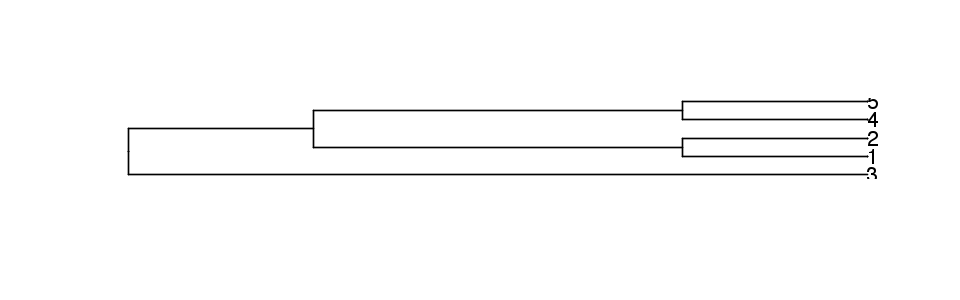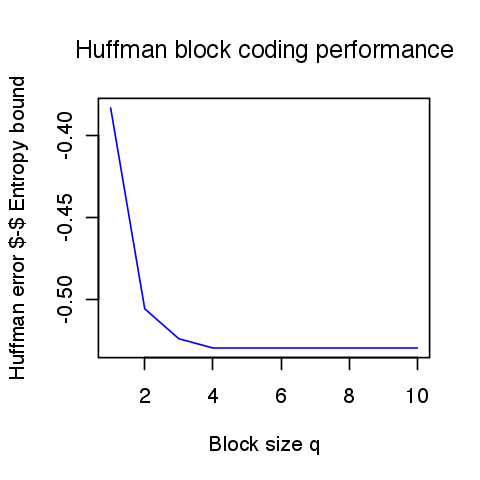# Entropic Coding and Compression¶

Important: Please read the installation page for details about how to install the toolboxes. $\newcommand{\dotp}{\langle #1, #2 \rangle}$ $\newcommand{\enscond}{\lbrace #1, #2 \rbrace}$ $\newcommand{\pd}{ \frac{ \partial #1}{\partial #2} }$ $\newcommand{\umin}{\underset{#1}{\min}\;}$ $\newcommand{\umax}{\underset{#1}{\max}\;}$ $\newcommand{\umin}{\underset{#1}{\min}\;}$ $\newcommand{\uargmin}{\underset{#1}{argmin}\;}$ $\newcommand{\norm}{\|#1\|}$ $\newcommand{\abs}{\left|#1\right|}$ $\newcommand{\choice}{ \left\{ \begin{array}{l} #1 \end{array} \right. }$ $\newcommand{\pa}{\left(#1\right)}$ $\newcommand{\diag}{{diag}\left( #1 \right)}$ $\newcommand{\qandq}{\quad\text{and}\quad}$ $\newcommand{\qwhereq}{\quad\text{where}\quad}$ $\newcommand{\qifq}{ \quad \text{if} \quad }$ $\newcommand{\qarrq}{ \quad \Longrightarrow \quad }$ $\newcommand{\ZZ}{\mathbb{Z}}$ $\newcommand{\CC}{\mathbb{C}}$ $\newcommand{\RR}{\mathbb{R}}$ $\newcommand{\EE}{\mathbb{E}}$ $\newcommand{\Zz}{\mathcal{Z}}$ $\newcommand{\Ww}{\mathcal{W}}$ $\newcommand{\Vv}{\mathcal{V}}$ $\newcommand{\Nn}{\mathcal{N}}$ $\newcommand{\NN}{\mathcal{N}}$ $\newcommand{\Hh}{\mathcal{H}}$ $\newcommand{\Bb}{\mathcal{B}}$ $\newcommand{\Ee}{\mathcal{E}}$ $\newcommand{\Cc}{\mathcal{C}}$ $\newcommand{\Gg}{\mathcal{G}}$ $\newcommand{\Ss}{\mathcal{S}}$ $\newcommand{\Pp}{\mathcal{P}}$ $\newcommand{\Ff}{\mathcal{F}}$ $\newcommand{\Xx}{\mathcal{X}}$ $\newcommand{\Mm}{\mathcal{M}}$ $\newcommand{\Ii}{\mathcal{I}}$ $\newcommand{\Dd}{\mathcal{D}}$ $\newcommand{\Ll}{\mathcal{L}}$ $\newcommand{\Tt}{\mathcal{T}}$ $\newcommand{\si}{\sigma}$ $\newcommand{\al}{\alpha}$ $\newcommand{\la}{\lambda}$ $\newcommand{\ga}{\gamma}$ $\newcommand{\Ga}{\Gamma}$ $\newcommand{\La}{\Lambda}$ $\newcommand{\si}{\sigma}$ $\newcommand{\Si}{\Sigma}$ $\newcommand{\be}{\beta}$ $\newcommand{\de}{\delta}$ $\newcommand{\De}{\Delta}$ $\newcommand{\phi}{\varphi}$ $\newcommand{\th}{\theta}$ $\newcommand{\om}{\omega}$ $\newcommand{\Om}{\Omega}$

This numerical tour studies source coding using entropic coders (Huffman and arithmetic).

You need to install the package ape

In :
options(repr.plot.width=3.5, repr.plot.height=3.5)
options(warn=-1) # turns off warnings, to turn on: "options(warn=0)"

library(Matrix)
library(stringr)
library(ape)

# Importing the libraries
for (f in list.files(path="nt_toolbox/toolbox_general/", pattern="*.R")) {
source(paste("nt_toolbox/toolbox_general/", f, sep=""))
}
for (f in list.files(path="nt_toolbox/toolbox_signal/", pattern="*.R")) {
source(paste("nt_toolbox/toolbox_signal/", f, sep=""))
}


## Source Coding and Entropy¶

Entropic coding converts a vector $x$ of integers into a binary stream $y$. Entropic coding exploits the redundancies in the statistical distribution of the entries of $x$ to reduce as much as possible the size of $y$. The lower bound for the number of bits $p$ of $y$ is the Shannon bound :

$$p=-\sum_ih(i)\log_2(h(i))$$

where $h(i)$ is the probability of apparition of symbol $i$ in $x$.

Fist we generate a simple binary signal $x$ so that $0$ has a probability $p$ to appear in $x$.

Probability of 0.

In :
p = 0.1


Size.

In :
n = 512


Signal, should be with token 1,2.

In :
x = ceiling(runif(n, 0, 1) > p ) +1


One can check the probabilities by computing the empirical histogram.

In :
h = c(sum(x==1), sum(x==2))
h = h/sum(h)

print(paste("Empirical p =" , h))

 "Empirical p = 0.083984375"


We can compute the entropy of the distribution represented as a vector $h$ of proability that should sum to 1. We take a max to avoid problems with null probabilties.

In :
e = - sum(h*log2(c(max(h,1e-20), max(h,1e-20))))
print(paste("Entropy = ", e))

 "Entropy =  0.416065091363473"


## Huffman Coding¶

A Hufman code $C$ associates to each symbol $i$ in $\{1,...,m\}$ a binary code $C_i$ whose length is as close as possible to the optimal bound $-\log_2\left(h(i)\right)$, where $h(i)$ is the probability of apparition of the symbol $i$.

We select a set of proabilities.

In :
h = c(.1, .15, .4, .15, .2)


The tree $T$ contains the codes and is generated by an iterative algorithm. The initial "tree" is a collection of empty trees, pointing to the symbols numbers.

In :
m = length(h)
T = list(list())


We build iteratively the Huffman tree by grouping together the two erees that have the smallest probabilities. The merged tree has a probability which is the sum of the two selected probabilities.

Initial probability.

In :
#we use the symbols i = 1,2,3,4,5 (as strings) with the associated probabilities h(i)

for (i in (1:m))
{
T[[i]] = list(toString(i), h[i])

}


Iterative merging of the leading probabilities.

In :
while (length(T)>=2)
{
T = T[order(sapply(T,'[[',2))]
q = as.numeric(T[])+as.numeric(T[])
t = T[1:2]
T = T[-(1:2)]
T[[length(T)+1]] = list(t,q)
}


We trim the computed tree by removing the probabilities.

In :
trim = function(T)
{
T0 = T[]
if (typeof(T0[]) == 'character')
{
return (T0)
}
else
{
return (list(trim(T0[]),trim(T0[])))
}
}

K = list()
K[] = list(trim(T)[][],trim(T)[])
T = K


We display T

In :
# flatten list
x2 = paste0(lapply(T, function(y) paste0("(", paste0(y, collapse = ","), ")")), collapse = ",")

# remove unwanted characters
x2 = gsub('\"|c|list| ', "", x2)
x2 = paste0("(", x2, ");")

# remove brackets from single term list object
x3 = str_replace_all(x2, "\$[a-z]*\$", function(x) gsub("^\$|\$$", "", x)) # plot plot(read.tree(text = x3), color="blue")Once the tree$T$is computed, one can compute the code$C_{i}$associated to each symbol$i$. This requires to perform a deep first search in the tree and stop at each node. In : codes = list() c = compute_huffcode(h) for (i in (1:length(h))) { codes[[toString(i)]] = c[i] }  Display the code. In : for (e in (1:length(codes))) { print(paste("Code of token", e, "=", codes[[toString(e)]])) }   "Code of token 1 = 100"  "Code of token 2 = 110"  "Code of token 3 = 0"  "Code of token 4 = 101"  "Code of token 5 = 111"  We draw a vector$x$according to the distribution$h$. Size of the signal. In : n = 1024  Randomization. In : rand_discr = function (p, m) { # rand_discr - discrete random generator # y = rand_discr(p, n); # y is a random vector of length n drawn from # a variable X such that # p(i) = Prob( X=i ) p = p/sum(p) n = length(p) coin = runif(m, 0, 1) cumprob = c(0,cumsum(p)) sample = matrix(0, nrow=1, ncol=m) for (j in (1:n)) { ind = c((coin > cumprob[j]) & (coin <= cumprob[j+1])) sample[ind] = j } return (sample) } x = rand_discr(h, n)  Exercise 1 Implement the coding of the vector$x$to obtain a binary vector$y$, which corresponds to replacing each sybmol$x(i)$by the code$C_{x(i)}$. In : source("nt_solutions/coding_2_entropic/exo1.R")  In : ## Insert your code here.  Compare the length of the code with the entropy bound. In : e = - sum(h*log2(c(max(h,1e-20),max(h,1e-20),max(h,1e-20),max(h,1e-20),max(h,1e-20)))) print(paste("Entropy bound = ", n*e)) print(paste("Huffman code = ", nchar(y)))   "Entropy bound = 2197.95388894312"  "Huffman code = 2256"  Decoding is more complicated, since it requires to iteratively parse the tree$T$. Initial empty decoded stream. In : x1 = c()  Perform decoding. In : T0 = K for (e in strsplit(y,split='')) { if (e == '0') { T0 = T0[] } else { T0 = T0[][] } if (typeof(T0) == 'character') { i = i+1 x1 = c(x1,T0) T0 = T } }  We test if the decoding is correct. In : err = norm(x - as.double(x1)) print(paste("Error (should be zero) : ", err))   "Error (should be zero) : 0"  ## Huffman Block Coding¶ A Huffman coder is inefficient because it can distribute only an integer number of bit per symbol. In particular, distribution where one of the symbol has a large probability are not well coded using a Huffman code. This can be aleviated by replacing the set of$m$symbols by$m^q$symbols obtained by packing the symbols by blocks of$q$(here we use$m=2$for a binary alphabet). This breaks symbols with large probability into many symbols with smaller proablity, thus approaching the Shannon entropy bound. Generate a binary vector with a high probability of having 1, so that the Huffman code is not very efficient (far from Shanon bound). Proability of having 0. In : t = .12  Probability distriution. In : h = c(t, 1-t)  Generate signal. In : n = 4096 * 2 x = (runif(n, 0, 1) >t) +1  For block of length$q=3$, create a new vector by coding each block with an integer in$\{1,...,m^q=2^3\}$. The new length of the vector is$n_1/q$where$n_1=\lceil n/q\rceil q$. Block size. In : q = 3  Maximum token value. In : m = 2  New size. In : n1 = (floor(n/q)+1)*q  New vector. In : x1 = matrix(0, nrow=1, ncol=n1) x1[1:length(x)] = x x1[length(x):length(x1)] = 1 x1 = x1 - 1 x2 = c() for (i in seq(1,n1,by=q)) { mult = m**c(1:q-1) x2 = c(x2, sum(x1[i:(i+q)]*mult)) }  We generate the probability table$H$of$x_1$that represents the probability of each new block symbols in$\{1,...,m^q\}$. In : H = h for (i in (1:(q-1))) { Hold = H H = c() for (j in (1:length(h))) { H = c(H, h[j]*Hold) } }  A simpler way to compute this block-histogram is to use the Kronecker product. In : H = h for (i in (1:(q-1))) { H = kronecker(H,h) } H  1. 0.001728 2. 0.012672 3. 0.012672 4. 0.092928 5. 0.012672 6. 0.092928 7. 0.092928 8. 0.681472 Exercise 2 For various values of block size$k\$, Perform the Huffman coding and compute the length of the code. Compare with the entropy lower bound.

In :
source("nt_solutions/coding_2_entropic/exo2.R")

 "Entropy Bound = 0.529360865287364"
 "---"
 "Huffman(block size =  2 = 0.1458740234375"
 "Huffman(block size =  3 = 0.0238037109375"
 "Huffman(block size =  4 = 0.005615234375"
 "Huffman(block size =  5 = 0"
 "Huffman(block size =  6 = 0"
 "Huffman(block size =  7 = 0"
 "Huffman(block size =  8 = 0"
 "Huffman(block size =  9 = 0"
 "Huffman(block size =  10 = 0"
 "Huffman(block size =  11 = 0"In :
## Insert your code here.